# Colleges with the highest SAT scores in Michigan

Top 10 colleges in Michigan with the highest SAT scores
Looking for the colleges with the highest SAT scores in Michigan? Well you're in luck! We've compiled a national college database and have created a list of the top 10 universities with the highest SAT scores in Michigan. These are the schools whose applicants had the highest average SAT scores in Michigan. And, since these tests are meant to determine academic prowess, they are arguably the schools with the most academically proficient students. You could even say these are the best colleges in Michigan. We also include each college's ACT scores and acceptance rate so that you can see where you would have the easiest or hardest time getting in. Read on to find out more.

## University of Michigan at Ann Arbor SAT scores

The average SAT score for University of Michigan at Ann Arbor is 1435.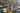The average SAT score of 1435 breaks down into:

• SAT math: 735

The average ACT score for University of Michigan at Ann Arbor is 33 and their acceptance rate is 22.9%.

## Hillsdale College SAT scores

The average SAT score for Hillsdale College is 1363.The average SAT score of 1363 breaks down into:

• SAT math: 670

The average ACT score for Hillsdale College is 31 and their acceptance rate is 36%.

## Kettering University SAT scores

The average SAT score for Kettering University is 1270.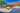The average SAT score of 1270 breaks down into:

• SAT math: 650

The average ACT score for Kettering University is 27 and their acceptance rate is 70.3%.

## Calvin College SAT scores

The average SAT score for Calvin College is 1265.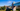The average SAT score of 1265 breaks down into:

• SAT math: 640

The average ACT score for Calvin College is 27 and their acceptance rate is 79%.

## Kalamazoo College SAT scores

The average SAT score for Kalamazoo College is 1260.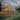The average SAT score of 1260 breaks down into:

• SAT math: 620

The average ACT score for Kalamazoo College is 28 and their acceptance rate is 72.8%.

## Michigan Technological University SAT scores

The average SAT score for Michigan Technological University is 1253.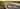The average SAT score of 1253 breaks down into:

• SAT math: 640

The average ACT score for Michigan Technological University is 27 and their acceptance rate is 74.5%.

## Aquinas College SAT scores

The average SAT score for Aquinas College is 1250.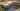The average SAT score of 1250 breaks down into:

• SAT math: 590

The average ACT score for Aquinas College is 24 and their acceptance rate is 51.9%.

## Hope College SAT scores

The average SAT score for Hope College is 1210.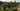The average SAT score of 1210 breaks down into:

• SAT math: 600

The average ACT score for Hope College is 27 and their acceptance rate is 84%.

## Michigan State University SAT scores

The average SAT score for Michigan State University is 1210.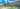The average SAT score of 1210 breaks down into:

• SAT math: 610

The average ACT score for Michigan State University is 26 and their acceptance rate is 71.1%.

## Andrews University SAT scores

The average SAT score for Andrews University is 1199.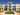The average SAT score of 1199 breaks down into:

• SAT math: 600